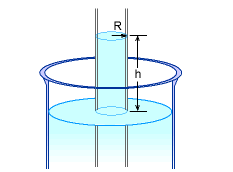Ch 1. Basics Multimedia Engineering Fluids MassDensity IdealGas Law Viscosity SurfaceTension VaporPressure
 Chapter 1. Basics 2. Fluid Statics 3. Kinematics 4. Laws (Integral) 5. Laws (Diff.) 6. Modeling/Similitude 7. Inviscid 8. Viscous 9. External Flow 10. Open-Channel Appendix Basic Math Units Basic Equations Water/Air Tables Sections Search eBooks Dynamics Fluids Math Mechanics Statics Thermodynamics Author(s): Chean Chin Ngo Kurt Gramoll ©Kurt GramollFLUID MECHANICS - CASE STUDY SOLUTIONCapillary Action The height of the water column is given as a function of the contact angle (θ), radius of the tube (R), density (ρ) and surface tension (σ) of the liquid as follows:      h = 2σcosθ / ρgR For water in contact with a clean glass, the contact angle is zero, hence the equation reduces to      h = 2σ / ρgR For water at 30oC, the surface tension and density are 0.0712 N/m and 995.7 kg/m3 respectively, as noted in the theory page. For a tube with a radius of 1 mm:      h = 2(0.0712)(106)/(995.7)(9.8)(1)        = 14.6 mm For a tube with a radius of 10 mm:      h = 2(0.0712)(106)/(995.7)(9.8)(10)        = 1.46 mm Note that the water rise in the capillary tube decreases with an increase of the radius of the tube.

Practice Homework and Test problems now available in the 'Eng Fluids' mobile app
Includes over 250 problems with complete detailed solutions.
Available now at the Google Play Store and Apple App Store.